Thursday, August 5, 2021
Home > NTSE > Mock Test Papers for NTSE Exam

# Mock Test Papers for NTSE Exam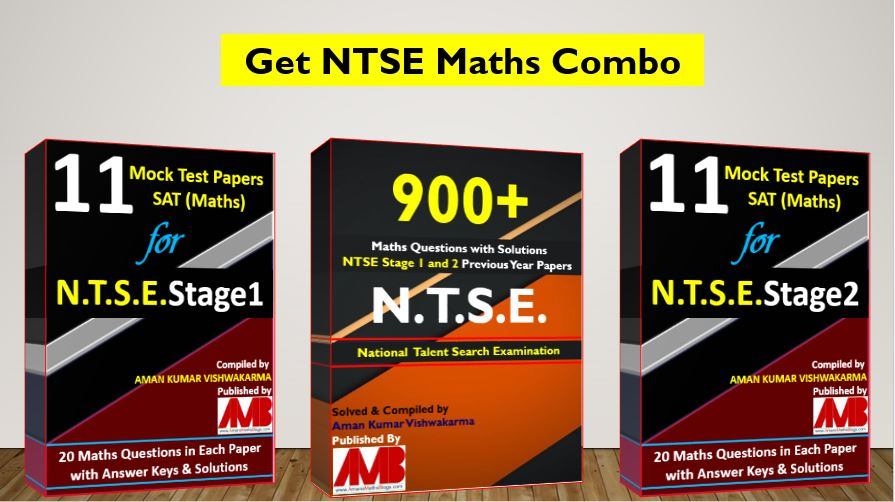Hi Students, welcome to Amans Maths Blogs (AMB). On this blog post, you will get the Mock Test Papers for NTSE Stage 1 and 2. These mock test papers are sample papers for NTSE Exams. As you know that, this maths blogs AMB is a special blogs for maths subjects. Thus, these mock test papers consisting only Maths questions, which help to practice for NTSE Stage 1 and 2 SAT (Maths) Papers.

# Mock Test Papers for NTSE Stage 1

I have complied 11 Mock Test Papers for NTSE Stage 1 consisting only Maths questions. Each Mock Test Papers for NTSE contains 20 maths questions along with answer keys and its solutions. All the questions are based on topics of Maths that is the syllabus of NTSE. To get the NTSE Stage 1 Mock Test Papers for SAT Maths, Click Here.

# Mock Test Papers for NTSE Stage 2

You are reading this post, it means you cleared the NTSE Stage 1 exam 2018-19, which was held on 04 Nov 2018. And, now you are preparing for NTSE Stage 2 Exam which will be scheduled on 16 June 2019 (Sunday). To know about the full details of NTSE Stage 1 and NTSE Stage 2 exam, click here.

In SAT (Maths) paper of NTSE Stage 1 and 2, the questions asked are of following Maths concepts. Arithmetic Progression, Circle, Congruent Triangles, Coordinate Geometry, Exponents, Height & Distance, Linear Equations, Logarithms, Mensuration, Number System, Polynomial, Probability, Quadratic Equations, Quadrilateral, Real Numbers, Shaded Regions, Similar Triangles, Statistics, Straight Lines, Tangent To Circle, Trigonometry.

Read More : 129 Maths Short Tricks

Thus, on this post, I have complied 11 Mock Test Papers for NTSE consisting only Maths questions. Each Mock Test Papers for NTSE contains 20 maths questions along with answer keys and its solutions. All the questions are based on the above topics of Maths that is the syllabus of NTSE.

## NTSE Mock Test Paper 1 : SAT (Maths)

Mock Test Paper for NTSE Stage 2 Question No 1:

A father has 4 children with at least two boys. The probability that he has 3 boys and a girl is

Options:

A. 1/2

B. 1/3

C. 1/4

D. 1/5

Mock Test Paper for NTSE Stage 2 Question No 2:

In the given figure, ABCD is a square. Find the value of tanx + tany.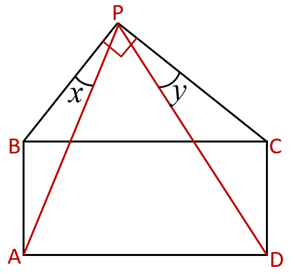Options:

A. 1/2

B. 2

C. 1/4

D. 1

Mock Test Paper for NTSE Stage 2 Question No 3:

If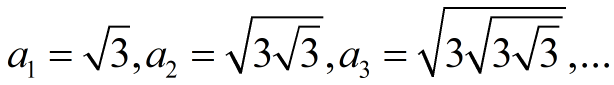and so on,then find the value of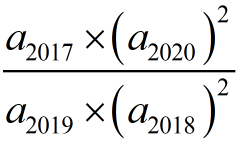Options:

A. 1

B. 2

C. 9

D. 16

Mock Test Paper for NTSE Stage 2 Question No 4:

Ifthen find the value of (xyz)102 – 1

Options:

A. 0

B. -1

C. 1

D. 2

Mock Test Paper for NTSE Stage 2 Question No 5:

In an AP, there are 25 terms. If t3 + t4 = 56, then find the sum of 25 terms of the AP.

Options:

A. 640

B. 720

C. 700

D. 540

Mock Test Paper for NTSE Stage 2 Question No 6:

Find the value of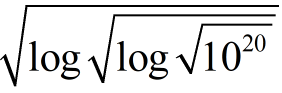Options:

A. Root(2)

B. 1/Root(2)

C. Root(16)

D. 1/Root(10)

Mock Test Paper for NTSE Stage 2 Question No 7:

The rational numbercan be written as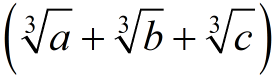, then find the value of (a + b + c)

Options:

A. 20

B. 21

C. 22

D. 23

Mock Test Paper for NTSE Stage 2 Question No 8:

If the roots of the equation x2 – mx + 4n = 0 are differ by 5, then

Options:

A. m2 = 16n + 25

B. m2 = 16n – 25

C. n2 = 25 – 16m

D. n2 = 16m – 25

Mock Test Paper for NTSE Stage 2 Question No 9:

If x + 1 = 1/2y and x – 1 = 1/y, where y0, then the value of x + y is

Options:

A. -3

B. -7

C. -13/4

D. -1/4

Mock Test Paper for NTSE Stage 2 Question No 10:

If a, b, c are three real numbers such that 3b – 10 = 7a + 2(c – 5), then the equation ax + by + c = 0 always passes through the point

Options:

A. (-7/2, 3/2)

B. (5/3, 7)

C. (7, 3)

D. (-7/2, 3/2)

Mock Test Paper for NTSE Stage 2 Question No 11:

In the figure, ΔABC and ΔCPD are congruent. The value of α is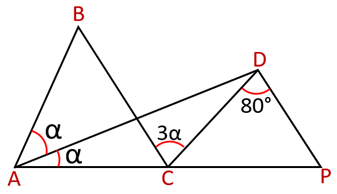Options:

A. 10 Degree

B. 15 Degree

C. 20 Degree

D. 25 Degree

Mock Test Paper for NTSE Stage 2 Question No 12:

In the figure, ABCD and PQD are squares. If CR = x and RD = y, then the value of TR in terms of x and y is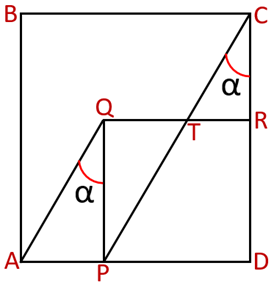Options:

A. x/y

B. x2/y

C. Root(xy)

D. (x + y)/2

Mock Test Paper for NTSE Stage 2 Question No 13:

In the figure, O is the centre of the square ABCD. If CN = 3 and BN = 8, then find the value of OM.Options:

A. 5.5

B. 6

C. 4.5

D. 7

Mock Test Paper for NTSE Stage 2 Question No 14:

In the given figure, then the value of x.Options:

A. 60 Degree

B. 55 Degree

C. 65 Degree

D. 45 Degree

Mock Test Paper for NTSE Stage 2 Question No 15:

In the given figure, ABCD is a quadrilateral circumscribed a circle. If the perimeter of the quadrilateral ABCD is 12 and AB = 1, then find the value of CD.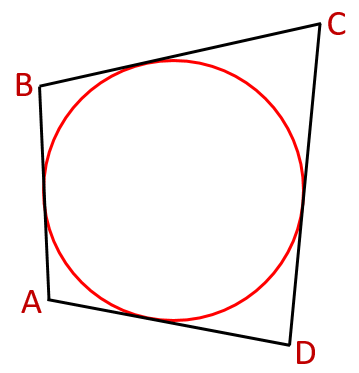Options:

A. 5

B. 6

C. 7

D. 8

Mock Test Paper for NTSE Stage 2 Question No 16:

In the given figure, if the area of the regular hexagon is A, then the area of the shaded region.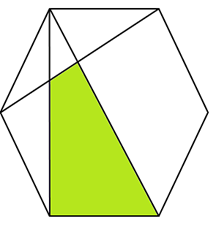Options:

A. A/3

B. 11A/36

C. 7A/24

D. 9A/22

Mock Test Paper for NTSE Stage 2 Question No 17:

If the HCF of A and 20 is 4, then find the sum of possible values of A, where A < 30.

Options:

A. 90

B. 92

C. 94

D. 98

Mock Test Paper for NTSE Stage 2 Question No 18:

In the given figure, find the value of x.Options:

A. r(sinθ + 1)/cosθ

B. r(cosθ – 1)/tanθ

C. r(cosecθ + 1)/cotθ

D. r/(1 – sinθ)

Mock Test Paper for NTSE Stage 2 Question No 19:

Find the volume of the solid generated by revolving with diagonal about a line L.Options:

A. 24π

B. 64π

C. 48π

D. 32π

Mock Test Paper for NTSE Stage 2 Question No 20:

Let ‘a’ be the midpoint and ‘b’ be the upper class limit of a class in a continuous frequency distribution. The lower limit of the class is

Options:

A. (2a + b)

B. (2b – a)

C. (2a – b)

D. 2(a + b)

The Answer Keys & Solutions of this NTSE Mock Test Paper 1 SAT (Maths) are in PDF file. To get this, click below RED button.Mock Test Paper for NTSE Stage 2 SAT (Maths): Paper 1 (FREE)

## NTSE Mock Test Paper 2: SAT (Maths)

The Question Paper and its Answer Keys & Solutions of this NTSE Mock Test Paper 2 SAT (Maths) are in PDF file. To get this, click below RED button.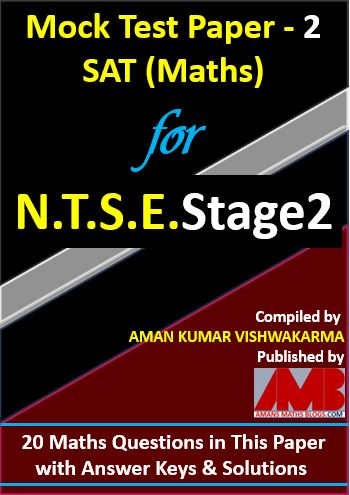Mock Test Paper for NTSE Stage 2 SAT (Maths): Paper 2 (Rs. 25)

## NTSE Mock Test Paper 3: SAT (Maths)

The Question Paper and its Answer Keys & Solutions of this NTSE Mock Test Paper 2 SAT (Maths) are in PDF file. To get this, click below RED button.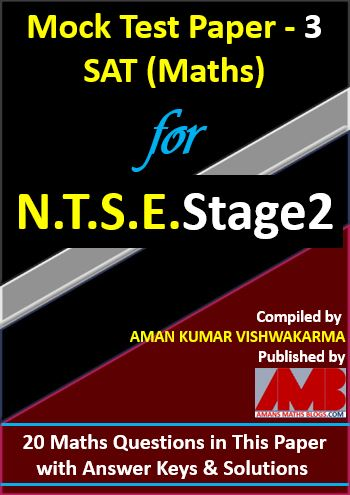Mock Test Paper for NTSE Stage 2 SAT (Maths): Paper 3 (Rs. 25)

## NTSE Mock Test Paper 4: SAT (Maths)

The Question Paper and its Answer Keys & Solutions of this NTSE Mock Test Paper 2 SAT (Maths) are in PDF file. To get this, click below RED button.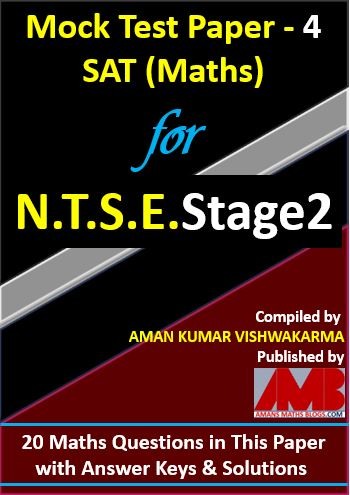Mock Test Paper for NTSE Stage 2 SAT (Maths): Paper 4 (Rs. 25)

## NTSE Mock Test Paper 5: SAT (Maths)

The Question Paper and its Answer Keys & Solutions of this NTSE Mock Test Paper 2 SAT (Maths) are in PDF file. To get this, click below RED button.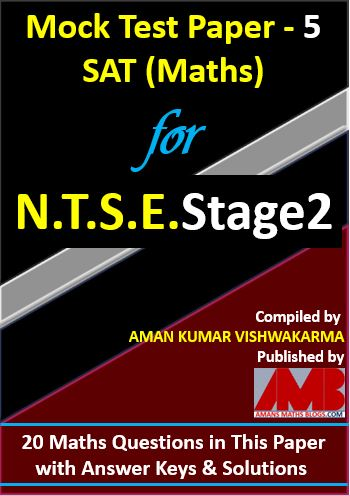Mock Test Paper for NTSE Stage 2 SAT (Maths): Paper 5 (Rs. 25)

## NTSE Mock Test Paper 6: SAT (Maths)

The Question Paper and its Answer Keys & Solutions of this NTSE Mock Test Paper 2 SAT (Maths) are in PDF file. To get this, click below RED button.Mock Test Paper for NTSE Stage 2 SAT (Maths): Paper 6 (Rs. 25)

## NTSE Mock Test Paper 7: SAT (Maths)

The Question Paper and its Answer Keys & Solutions of this NTSE Mock Test Paper 2 SAT (Maths) are in PDF file. To get this, click below RED button.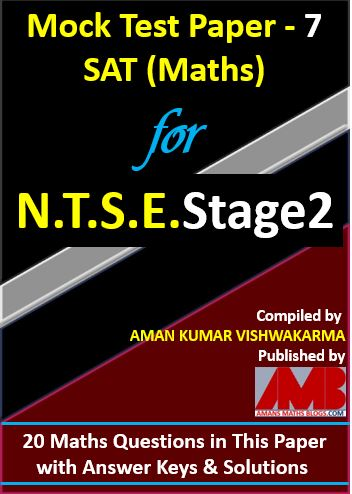Mock Test Paper for NTSE Stage 2 SAT (Maths): Paper 7 (Rs. 25)

## NTSE Mock Test Paper 8: SAT (Maths)

The Question Paper and its Answer Keys & Solutions of this NTSE Mock Test Paper 2 SAT (Maths) are in PDF file. To get this, click below RED button.Mock Test Paper for NTSE Stage 2 SAT (Maths): Paper 8 (Rs. 25)

## NTSE Mock Test Paper 9: SAT (Maths)

The Question Paper and its Answer Keys & Solutions of this NTSE Mock Test Paper 2 SAT (Maths) are in PDF file. To get this, click below RED button.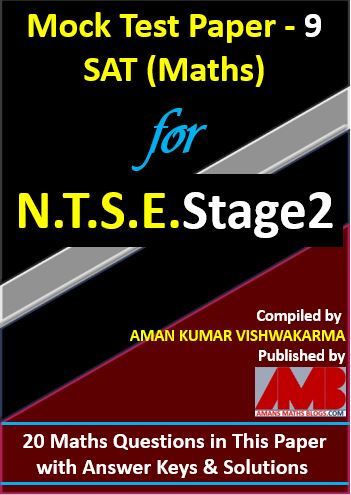Mock Test Paper for NTSE Stage 2 SAT (Maths): Paper 9 (Rs. 25)

## NTSE Mock Test Paper 10: SAT (Maths)

The Question Paper and its Answer Keys & Solutions of this NTSE Mock Test Paper 2 SAT (Maths) are in PDF file. To get this, click below RED button.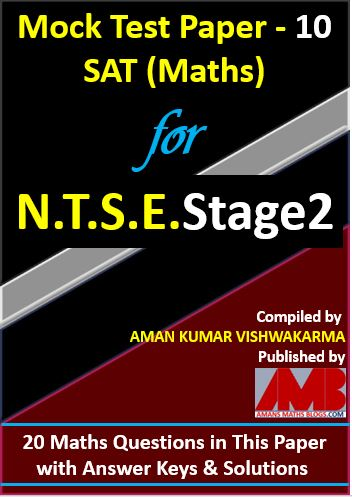Mock Test Paper for NTSE Stage 2 SAT (Maths): Paper 10 (Rs. 25)

## NTSE Mock Test Paper 11: SAT (Maths)

The Question Paper and its Answer Keys & Solutions of this NTSE Mock Test Paper 2 SAT (Maths) are in PDF file. To get this, click below RED button.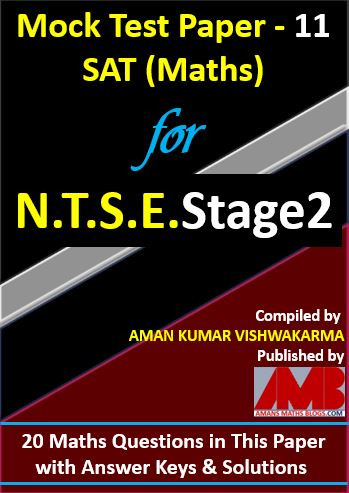Mock Test Paper for NTSE Stage 2 SAT (Maths): Paper 11 (Rs. 25)

## NTSE Mock Test Paper Combined (1 to 11): SAT (Maths)

The Question Paper and its Answer Keys & Solutions of this NTSE Mock Test Paper 2 SAT (Maths) are in PDF file. To get this, click below RED button.

Total cost of all 10 Mock Test Papers for NTSE Stage 2 is 10xRs25 = Rs 250. But if you get all 11 Mock Test Papers for NTSE Stage 2, you will get 52% off.11 Mock Test Paper for NTSE Stage 2 SAT (Maths) (52% Off)

## NTSE Maths Combo Pack EBook

Get NTSE Maths Previous Year Question Bank with 900+ Questions and its Solutions and 11 Mock Test Papers EBook. To get this combo ebook, click below RED button.900+ Math Questions Bank & 11 Mock Test Paper for NTSE SAT (Maths)

error: Content is protected !!